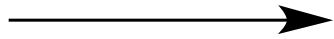Illustrated Glossary of Organic Chemistry

m/z (mass-to-charge ratio): In mass spectrometry the ratio of an ion's mass (m) in atomic mass units (amu) to its formal charge (z). Formal charge is usually +1. The units for m/z are usually not included.FragmentationCH3CH2CH2+ +Molecular ion Fragments Formula = C5H12O Mass = 88 amu Charge = +1 m/z = 88 Formula = C3H7 Mass = 43 amu Charge = +1 m/z = 43 Formula = C2H5O Mass = 45 amu Charge = +1 m/z = 45